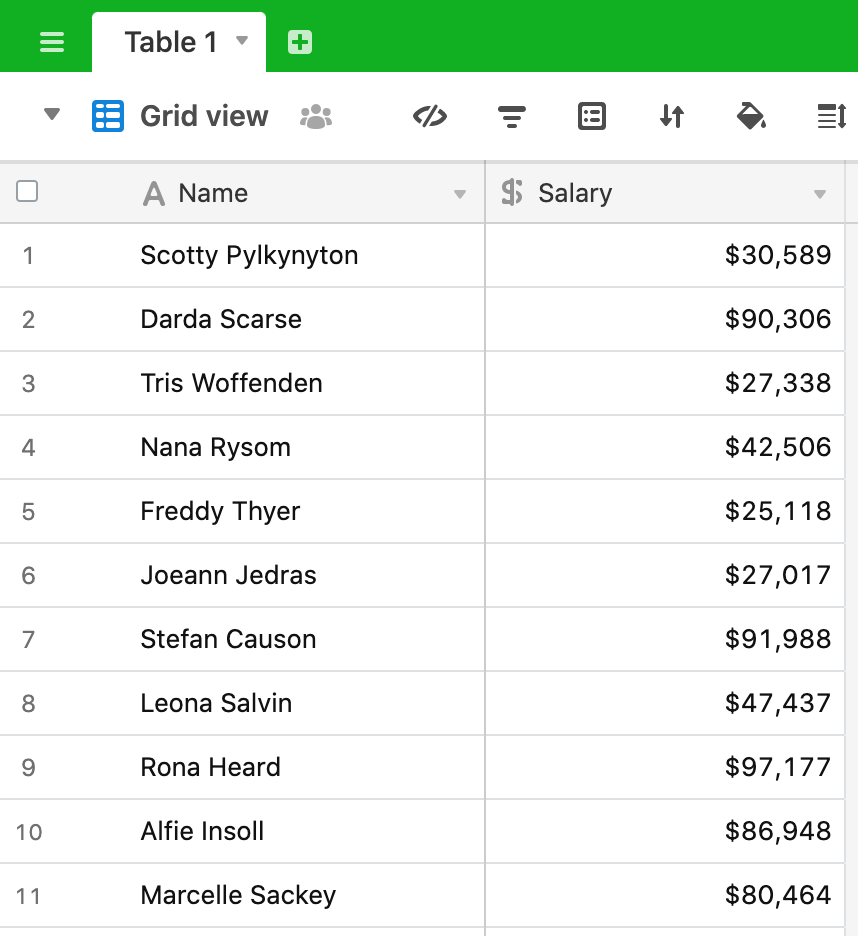# Calculating percentiles

A recent post on the Airtable community forum asked about calculating the kth percentile of a set of data. This is not easy to do in Airtable using formulas, linked records and lookups but is a perfect use case for an Airtable script. So, here we go:

Let’s say we’ve got a set of data which shows a number of people and the salary they earn:We want to calculate the kth percentile of the data set. From here, this is defined as:

The kth percentile is a value in a data set that splits the data into two pieces: The lower piece contains k percent of the data, and the upper piece contains the rest of the data (which amounts to [100 – k] percent, because the total amount of data is 100%).

We’re going to follow the process outlined at the link above, specifically:

Step 1: Order all the values in the data set from smallest to largest.
Step 2: Multiply k percent by the total number of values, n. This number is called the index.
Step 3: If the index obtained in Step 2 is not a whole number, round it up to the nearest whole number and go to Step 4a. If the index obtained in Step 2 is a whole number, go to Step 4b.
Step 4a: Count the values in your data set from left to right (from the smallest to the largest value) until you reach the number indicated by Step 3. The corresponding value in your data set is the kth percentile.
Step 4b: Count the values in your data set from left to right until you reach the number indicated by Step 2. The kth percentile is the average of that corresponding value in your data set and the value that directly follows it.

Here’s our script:

``````
let table = base.getTable('Table 1');
let query = await table.selectRecordsAsync();
let percentileInput = await input.textAsync('Please enter the percentile you want to calculate (must be a number between 0 and 100');
let k = parseInt(percentileInput)/100;
let salaries = query.records.map(record => {
return record.getCellValue('Salary')
})

let ordered_salaries = salaries.sort();

let n = ordered_salaries.length;
let index = Math.ceil(k * n);
let result;

if (index != (k*n)) {
result = ordered_salaries[index-1];
} else {
result = (ordered_salaries[index-1] + ordered_salaries[index])/2;
}

output.text(`The \${percentileInput} percentile is \${result}`);

``````

Let’s break this down.

We start by defining the table and query as we often do:

``````
let table = base.getTable('Table 1');
let query = await table.selectRecordsAsync();

``````

The next line asks the user to input the percentile they want to calculate - 50th, 75th and so on:

``````
let percentileInput = await input.textAsync('Please enter the percentile you want to calculate (must be a number between 0 and 100');

``````

Note: the script asks them to input a number between 0 and 100. Arguably, we could validate the input here to ensure that it is 1) a number and 2) between 0 and 100, but let’s leave that for another post 😉.

The next line of the script is:

``````
let k = parseInt(percentileInput)/100;

``````

Here, we take the (string) input, covert it to an integer and divide it by 100 so that it is represented as a percentage value. We then use `map` to extract the set of salary values to a new array:

``````
let salaries = query.records.map(record => {
return record.getCellValue('Salary')
})

``````

Everything we’ve done in the script to this point is just preparation for the percentile calculation. The next line of the script completes Step 1 of the process, sorting the values from smallest to largest:

``````
let ordered_salaries = salaries.sort();

``````

In Step 2 we multiply `k` by `n` (the number of observations). `n` is the length of the array of salaries, so we get the value here:

``````
let n = ordered_salaries.length;

``````

Here we take a slight deviation from the exact method described by calcuating `k * n` and rounding this up using the function `Math.ceil`. This gives us a whole number value for the variable `index`.

We now compare `index` to `k * n` to determine if we do the “non-whole number” step (Step 4a) or the “whole number” step (Step 4b).

``````
if (index != (k*n)) {
result = ordered_salaries[index-1];
} else {
result = (ordered_salaries[index-1] + ordered_salaries[index])/2;
}

``````

So, if `index` is not equal to `k*n`, then `k * n` is not a whole number so we get the value from the salaries array that matches our `index - 1`. Note that we minus 1 from the index value because the array is zero-indexed, i.e. starts with index of zero. For more information on accessing elements of an array by the index, see here.

If `index` does equal `k * n` then `k * n` is a whole number and in this case, we get the average of the two values specified by the `index`.

Finally, we output the value for the chosen percentile:

``````
output.text(`The \${percentileInput} percentile is \${result}`);

``````